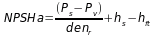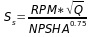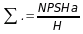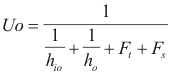Questions possible

What is the NPSHa?

NPSHA = net positive suction head availableif you suppose the vapors pressure(P v) is equal like suction pressure(Ps), then

NPSHa must be equal to the pressure inlet in feet of pumped liquid.; Suction specific speed.

Ss must be less of 10 000 RPM, and some special designs can operate up to 12 000 RPM, but no more.; cavitation coefficient.

H: is the differential head of the first stage.

What is the NPSH required?

This is a critical number of pump. It is used to prevent cavitation. This data is from the pump manufacturer.

NPSHa >1.1 NPSHr for hydrocarbons

NPSHa >1.2 NPSHa for water

Do you want how you can calculate this number?

What is CV?

Cv is number of character valve.

Cv is the number of gallons per minute of water that will pass through a given flow restriction with a pressure drop of one psi.

What is Damper?

It is a king of valve, which regulates the exit of gases for the chimney (pressure difference between air intake and air exit). See graphics.

The modern furnace is equipped with a lot of dampers(2 to 4). Their purpose is to regulate the amount of air reaching the fire and thus control the rate at which the fire burns.

What unit is used for fouling factor?

The fouling factor is in hr.sq.ft/Btu.

See this formula :Where             Uo is the overall coefficient of heat transfer (Btu/hr.sq.ft).

hio is the coefficient of heat transfer inside the tube (Btu/hr.sq.ft).

ho is the coefficient of heat transfer inside the shell (Btu/hr.sq.ft).

Ft is the fouling factor inside the tube(hr.sq.ft/Btu.).

Fs is the fouling factor inside the shell(hr.sq.ft/Btu).

For flowing cooling water, a fouling factor of 0.003 is used. For clean liquids and clean condensing vapors, a fouling factor of 0.001 is used.

BACK